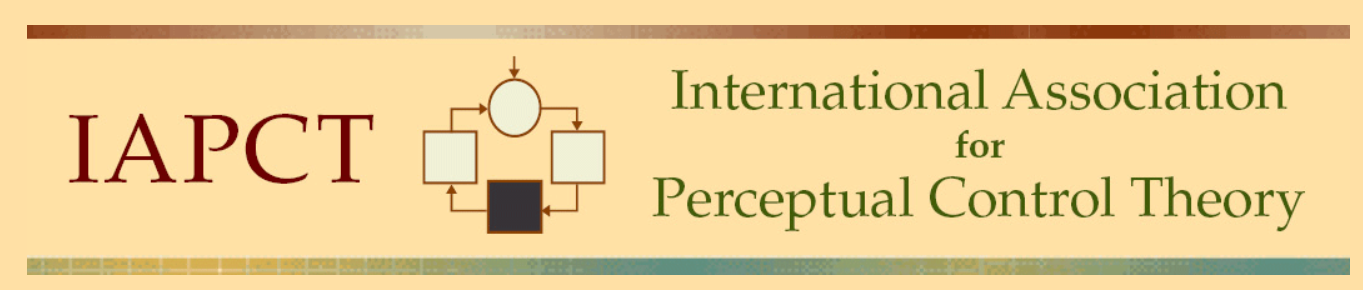# Goodness of fit

[From Bruce Abbott (961128.1025 EST)]

Bill Powers (961128.0130 MST) --

RMS error has to be compared with something, doesn't it? What about RMS
error divided by mean value? This would give us the error as a fraction of
the mean observed value. Of course that wouldn't work too well if the mean
observed value were close to zero.

RMS error basically tells you how far the the data are from the model's
predictions, on average, in standard deviation units. A given RMS error can
reflect a variety of faults in the model (not to mention noise in the data).
A large RMS error might occur if:

1. the prediction tracks the data perfectly but with a constant offset;
this constant offset would appear as a difference between the means of
the predicted and actual values.

2. the means of the two waveforms vary according to the same pattern and
have the same mean, but differing amplitudes.

3. the two waveforms have identical patterns, amplitudes, and means, but
differ in phase.

4. the two wave forms have identical amplitudes and means, and nearly
identical waveforms except for a very low-frequency component
(i.e., a slow drift).

5. the two wave forms have identical means and amplitudes but different
waveforms.

RMS error tells you how much of a problem you have with fit, but it does not
tell you what the source of that error is.

The correlation coefficient (Pearson r) removes any average difference
between the predicted and observed values (DC component), and then removes
any difference in rms amplitude between the waveforms. It thus compares the
shapes of the two waveforms. However, if there is a low-frequency component
of relatively large amplitude in comparison to higher-frequency components,
the size of Pearson r will mainly reflect the agreement between model and
data in the low-frequency component. For example, if model and data both
drift upward over time, and this upward drift accounts for most of the
change in Y over time, then the correlation will be high even though the
match with the higher-frequency components is poor.

In addition, Pearson r will be strongly affected by phase differences
between the two waveforms. A solution to this problem is to shift one
waveform with respect to the other by steps and examine the fit at each
step, a technique called cross correlation.

It would seem to me that a variety of measures are needed to adequately
assess the fit of model to data with respect to all these different aspects
of the two waveforms. I'm not sure how well it would work, but it would
seem that an analysis of the two waveforms into different frequency
components (e.g., by passing them through a low-pass filter, high-pass
filter, etc.) and then comparison of these filtered model and observed
waveforms might identify where the model fits best and worst (e.g., it might
do an excellent job of following the low-frequency variations in the data
while failing to reproduce the higher-frequency components).

Regards,

Bruce